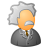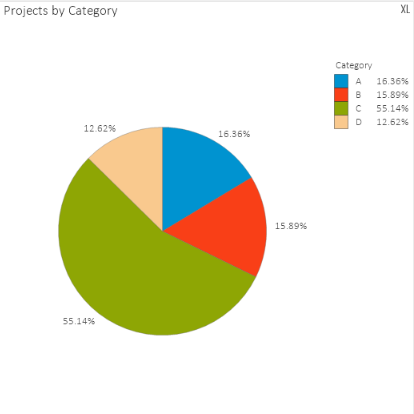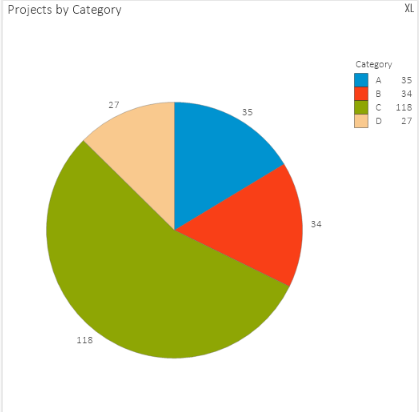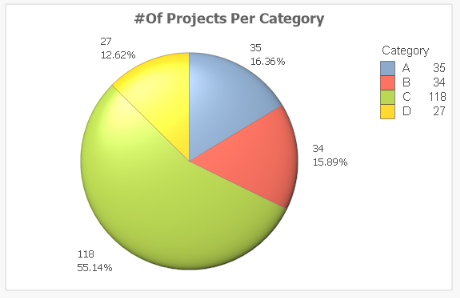cancel
Showing results for
Did you mean:Creator III

## How to show Count and Percentage on the Pie chart visual ?

Hello,

I have the below Pie Chart which shows the No of Projects per Category;When I uncheck the relative under my expression I am getting the count of the projects as shown below;How Can I get both percentages and count of the projects in my Pie chart visual? any help is greatly appreciated.

thanks

Bhavesh

1 Solution

Accepted SolutionsCreator III

Couple of answers that work here, I find the easiest option to be to simply add a second expression with the exact same expression (for example Count(Project)), and tick relative for one of the expressions and leave it off for the other. Check Value on data points for both and you should have what you want. Example:You won't be able to see the percentage in the legend though, you'll have to use Nassim's solution for that.

5 RepliesPartner - Master III

You need to use dual function.

something like

dual( your_sum_expression & '  - ' & your_percent_expression,your_sum_expression)

something like

dual(Num(Sum(Sales),'0,000.00') & '-' & num((Sum(Sales)/Sum(TOTAL Sales))*100,'0.00') & '%',

Num(Sum(Sales),'0,000.00'))Contributor III

Hi,

just add a calculated dimension  as

=Category&' : '& aggr(num(sum(#Projects)/sum(total{<Category>} #Projects),'#,##0.00%'),Category)

and the expression is :

sum(#Projects)

and don't select relative check box .

thanks

nassimCreator III

Couple of answers that work here, I find the easiest option to be to simply add a second expression with the exact same expression (for example Count(Project)), and tick relative for one of the expressions and leave it off for the other. Check Value on data points for both and you should have what you want. Example:You won't be able to see the percentage in the legend though, you'll have to use Nassim's solution for that.Creator III
Author

That was the easiest thing to do. thank youContributor

can this be expanded to a second dimension? so not just "category", but say, "category" and "projectowner" where project owner could be a list of namesCommunity Browser